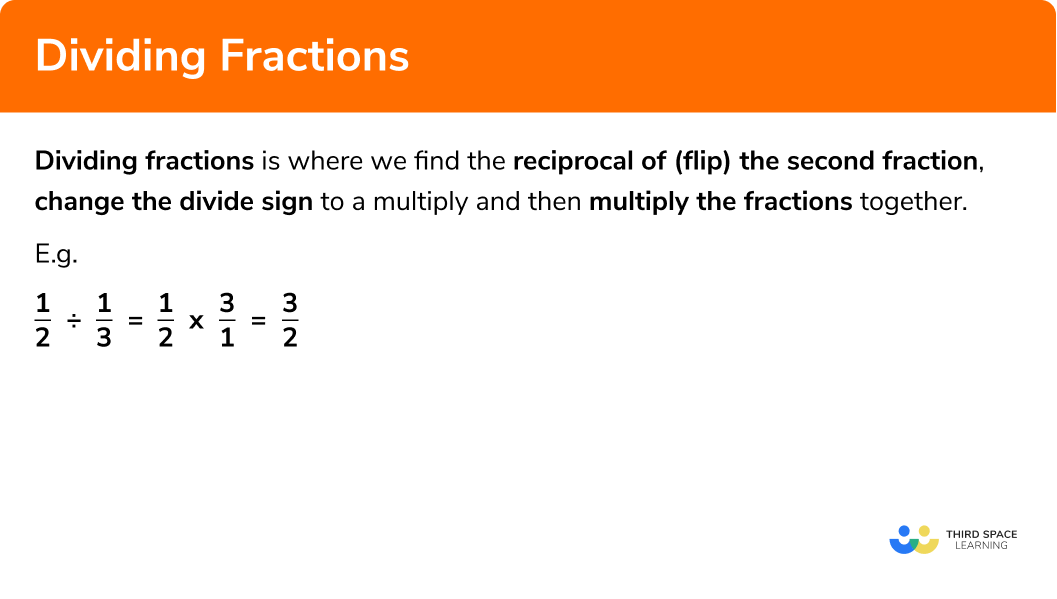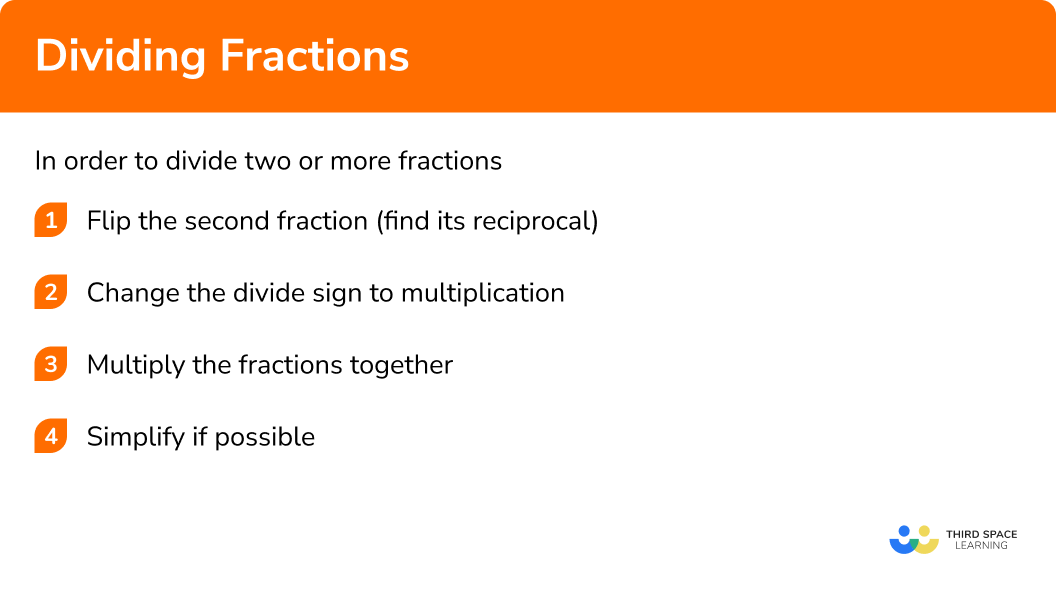# Dividing Fractions

Here we will learn about dividing fractions including how to divide fractions, divide fractions by whole numbers, and divide mixed fractions.

There are also dividing fractions worksheets based on Edexcel, AQA and OCR exam questions, along with further guidance on where to go next if you’re still stuck.

## What is dividing fractions?

Dividing fractions is where we find the reciprocal of (flip) the second fraction, change the divide sign to a multiply and then multiply the fractions together.

For example:

There are 6 pizzas eaten at a party. Each person eats half a pizza. How many people ate pizzas?

We need to find how many halves there are in 6 pizzas.

Let’s illustrate this with a diagram:

There are 12 halves so there must have been 12 people at the party.

So,

$6 \div \frac{1}{2}=12$

We notice that this is the same as 6 × 2 which equals 12.

So to divide two fractions we flip the second fraction and multiply them.

$\begin{array}{l} 6 \div \frac{1}{2} \\\\ 6 \times \frac{2}{1} \\\\ =12 \text { people } \end{array}$

### What is dividing fractions?## How to divide fractions

In order to divide fractions:

1. Flip the second fraction (find its reciprocal)
2. Change the divide sign to multiplication
3. Multiply the fractions together
4. Simplify if possible

Example: Divide two proper fractions

$\frac{1}{2} \div \frac{1}{3}$

Flip the second fraction:

$\frac{1}{2} \bigcirc\frac{3}{1}$

Multiply:

$\frac{1}{2} \times \frac{3}{1}=\frac{3}{2}$

Simplify if possible:

$\frac{3}{2}$

Example: Divide a fraction by a whole number

$\frac{1}{2} \div 4$

Put the whole number over 1:

$\frac{1}{2} \div \frac{4}{1}$

Flip the second fraction:

$\frac{1}{2} \bigcirc \frac{1}{4}$

Multiply:

$\frac{1}{2} \times \frac{1}{4}=\frac{1}{8}$

Simplify if possible:

$\frac{1}{8}$

Example: Divide two mixed number fractions

$1 \frac{1}{2} \div 2 \frac{1}{4}$

Change the mixed fractions into improper fractions:

$\frac{3}{2} \div \frac{9}{4}$

Flip the second fraction:

$\frac{3}{2} \bigcirc \frac{4}{9}$

Multiply:

$\frac{3}{2} \times \frac{4}{9}=\frac{12}{18}$

Simplify if possible:

$\frac{2}{3}$

### Explain how to divide fractions in 4 steps## What is a reciprocal?

The reciprocal of a number is a number which, when multiplied by the number itself, equals 1.

For example:

$\frac{2}{1} \times \frac{1}{2}= 1$

So,

$\frac{1}{2}$

is the reciprocal of

$\frac{2}{1}$

### Related lessons on fractions

Dividing fractions is part of our series of lessons to support revision on fractions. You may find it helpful to start with the main fractions lesson for a summary of what to expect, or use the step by step guides below for further detail on individual topics. Other lessons in this series include:

## Dividing fractions examples

### Example 1: dividing two proper fractions

Divide the fractions below:

$\frac{1}{7} \div \frac{1}{2}$

1. Flip the second fraction (find its reciprocal)

$\frac{1}{7} \bigcirc \frac{2}{1}$

Turn the second fraction upside down. The numerator becomes the denominator and vice versa.

2Change the divide sign to multiplication

$\frac{1}{7} \times \frac{2}{1}$

3Multiply the fractions together

$\frac{1}{7} \times \frac{2}{1}$

Remember: To multiply two fractions together multiply the numerators together and the denominators together.

$\frac{1 \times 2}{7 \times 1}=\frac{2}{7}$

4Simplify if possible

The fraction cannot be simplified so we are left with

$\frac{2}{7}$

### Example 2: dividing three proper fractions

Divide the fractions below:

$\frac{1}{5} \div \frac{1}{2} \div \frac{2}{3}$

In this case we have three fractions so flip the third fraction as well (find its reciprocal).

$\frac{1}{5} \bigcirc \frac{2}{1} \bigcirc \frac{3}{2}$

Turn the second and third fractions upside down. The numerator becomes the denominator and vice versa.

$\frac{1}{5} \times \frac{2}{1} \times \frac{3}{2}$

$\frac{1}{5} \times \frac{2}{1} \times \frac{3}{2}$

Remember: To multiply two fractions together multiply the numerators together and the denominators together.

$\frac{1 \times 2 \times 3}{5 \times 1 \times 2}=\frac{6}{10}$

$\frac{6}{10}$

can be simplified to

$\frac{6 \div 2}{10 \div 2}=\frac{3}{5}$

## How to divide fractions by whole numbers

In order to divide fractions by whole numbers:

1. Put the whole number over 1
2. Flip the second fraction (find its reciprocal)
3. Change the divide sign to multiplication
4. Multiply the fractions together
5. Simplify if possible

## Dividing fractions by whole numbers examples

### Example 3: dividing a fraction by a whole number

Divide the fractions below:

$\frac{1}{3} \div \ 4$
$\frac{1}{3} \div \frac{4}{1}$

$\frac{1}{3} \bigcirc \frac{1}{4}$

Turn the second fraction upside down. The numerator becomes the denominator and vice versa.

$\frac{1}{3} \times \frac{1}{4}$

$\frac{1}{3} \times \frac{1}{4}$

Remember: To multiply two fractions together multiply the numerators together and the denominators together.

$\frac{1 \times 1}{3 \times 4}=\frac{1}{12}$

The fraction cannot be simplified so we are left with

$\frac{1}{12}$

### Example 4: worded question dividing with a whole number

There are 12 pies eaten at a party. Each person ate a quarter of a pie. How many people ate pies?

First we need to create an equation to model the problem

Since 12 pies are eaten and each person ate a quarter, if we find out how many quarters there are in 12, it will tell us how many people ate pies.
We need to divide \frac{1}{4} into 12.

As an equation:

$12 \div \frac{1}{4}$

Now we are ready to perform the calculation:

$\frac{12}{1} \div \frac{1}{4}$

$\frac{12}{1} \bigcirc \frac{4}{1}$

Turn the second fraction upside down. The numerator becomes the denominator and vice versa.

$\frac{12}{1} \times \frac{4}{1}$

$\frac{12}{1} \times \frac{4}{1}$

Remember: To multiply two fractions together multiply the numerators together and the denominators together.

$\frac{12 \times 4}{1 \times 1}=\frac{48}{1}$

We are left with 48.

48 people ate pies.

## How to divide mixed fractions

In order to divide mixed fractions:

1. Change mixed fraction(s) to improper fraction(s)
2. Flip the second fraction (find its reciprocal)
3. Change the divide sign to multiplication
4. Multiply the fractions together
5. Simplify and convert back to a mixed number if possible

## Dividing mixed fractions examples

### Example 5: dividing mixed fractions

Divide the fractions below:

$1 \frac{1}{3} \div 2 \frac{1}{2}$

Remember: To convert a mixed number into an improper fraction multiply the denominator by the whole number and then add it to the numerator.

$1 \frac{+1}{\times3}=\frac{4}{3}$
$2 \frac{+1}{\times2}=\frac{5}{2}$

$\frac{4}{3} \bigcirc \frac{2}{5}$

Turn the second fraction upside down. The numerator becomes the denominator and vice versa.

$\frac{4}{3} \times \frac{2}{5}$

$\frac{4}{3} \times \frac{2}{5}$

Remember: To multiply two fractions together multiply the numerators together and the denominators together.

$\frac{4 \times 2}{3 \times 5}=\frac{8}{15}$

The fraction cannot be simplified so we are left with

$\frac{8}{15}$

### Example 6: worded question with dividing mixed fractions

A rectangle has an area of   1 \frac{1}{4} m^{2} and a width of \frac{1}{5} m . What is the length of the rectangle?

First we need to create an equation to model the problem

If we divide the width into the total area we will be able to calculate the length.

$l=1 \frac{1}{4} \div \frac{1}{5}$

Now we are ready to perform the calculation:

Remember: To convert a mixed number into an improper fraction multiply the denominator by the whole number and then add it to the numerator.

$1 \frac{+1}{\times4}=\frac{5}{4}$

$\frac{5}{4} \bigcirc \frac{5}{1}$

Turn the second fraction upside down. The numerator becomes the denominator and vice versa.

$\frac{5}{4} \times \frac{5}{1}$

$\frac{5}{4} \times \frac{5}{1}$

Remember: To multiply two fractions together multiply the numerators together and the denominators together.

$\frac{5 \times 5}{4 \times 1}=\frac{25}{4}$

The fraction cannot be simplified so we are left with a length of

$\frac{25}{4}m= 6 \frac{1}{4} \mathrm{m}$

### Common misconceptions

• Thinking that dividing by \frac{1}{2} is the same as halving a number

E.g.

$4 \div \frac{1}{2} \neq 2$

• Flipping the first fraction instead of the second fraction

A common error is to flip the first fraction instead of the second
E.g.

$\frac{9}{10} \div \frac{3}{5}$
$\frac{10}{9} \div \frac{3}{5}$

This is incorrect.

### Practice dividing fractions questions

1. \frac{4}{11} \div \frac{1}{7}

\frac{4}{77}\frac{17}{77}\frac{28}{11}\frac{81}{11}Flip the second fraction and change divide to multiply:

\begin{aligned} \frac{4}{11} \div \frac{1}{7} &= \frac{4}{11} \times \frac{7}{1}\\\\ &=\frac{28}{11} \end{aligned}

2. \frac{2}{3} \div \frac{6}{10}

\frac{10}{9}\frac{12}{18}\frac{21}{9}\frac{9}{10}Flip the second fraction and change divide to multiply:

\begin{aligned} \frac{2}{3} \div \frac{6}{10} &= \frac{2}{3} \times \frac{10}{6}\\\\ &= \frac{20}{18} \end{aligned}

Simplify the fraction: \frac{20}{18} = \frac{10}{9}

3. \frac{2}{9} \div \ 5

\frac{0.4}{1.8}\frac{10}{9}\frac{10}{45}\frac{2}{45}First we need to rewrite 5 as \frac{5}{1} .

We need to calculate \frac{2}{9} \div \frac{5}{1} .

Flip the second fraction and change divide to multiply:

\begin{aligned} \frac{2}{9} \div \frac{5}{1} &= \frac{2}{9} \times \frac{1}{5}\\\\ &=\frac{2}{45} \end{aligned}

4. A seamstress has 15 yards of fabric on the roll. She needs \frac{1}{3} yard of fabric to sew each garment. How many garments can she make?

5451812We need to calculate 15 \div \frac{1}{3} .

First we rewrite 15 as \frac{15}{1} and then flip the second fraction and change the divide to multiply.

\begin{aligned} \frac{15}{1} \div \frac{1}{3} &= \frac{15}{1} \times \frac{3}{1}\\\\ &= \frac{45}{1} \end{aligned}

\frac{45}{1} is 45 so she can make 45 garments.

5. 3 \frac{2}{3} \div 1 \frac{1}{5}

3 \frac{1}{18}6 \frac{1}{3}3 \frac{10}{3}4 \frac{6}{15}First we need to write the mixed numbers as improper fractions.

3 \frac{2}{3} = \frac{11}{3}\\

1 \frac{1}{5} = \frac{6}{5}

We need to calculate \frac{11}{3} \div \frac{6}{5} .

Flip the second fraction and change the divide to multiply:

\begin{aligned} \frac{11}{3} \div \frac{6}{5} &= \frac{11}{3} \times \frac{5}{6}\\\\ &= \frac{55}{18} \end{aligned}

Convert the fraction back to a mixed number: \frac{55}{18} = 3 \frac{1}{18}

6. There are 5 \frac{1}{3} pizzas at a party. Each person ate 1 \frac{1}{3} pizzas. How many people ate pizzas?

6543We need to calculate 5 \frac{1}{3} \div 1 \frac{1}{3} .

First we write the improper fractions as mixed numbers:

5 \frac{1}{3} = \frac{16}{3}

1 \frac{1}{3} = \frac{4}{3}

We need to calculate \frac{16}{3} \div \frac{4}{3} .

Flip the second fraction and change the divide to multiply:

\begin{aligned} \frac{16}{3} \div \frac{4}{3} &= \frac{16}{3} \times \frac{3}{4}\\\\ &= \frac{48}{12} \end{aligned}

Simplify the fraction: \frac{48}{12}=4

### Dividing fractions GCSE questions

1. Work out \frac{3}{5} \div \frac{1}{7}

(3 marks)

\frac{7}{1}

Evidence of flipping second fraction

(1)

Evidence of multiplication (multiplication sign seen)

(1)

\frac{21}{5}

(1)

2. Work out 1 \frac{1}{4} \div 2 \frac{2}{5} . Express your answer in the simplest form.

(4 marks)

\frac{5}{4} or \frac{12}{5} seen (converts to improper fraction)

(1)

Evidence of flipping the second fraction

(1)

Evidence of multiplication

(1)

\frac{25}{48}

(1)

3. (a) There are 24 pizzas eaten at a party. Each friend eats \frac{2}{3} of a pizza. How many friends were at the party?

(4 marks)

24 \div \frac{2}{3}

(1)

Evidence of finding the reciprocal of the second fraction \frac{3}{2} AND changing the divide to a multiply.

(1)

36 friends

(1)

## Learning checklist

You have now learned how to:

• Use the multiplication operation, including formal written methods, applied to proper and improper fractions and mixed numbers

## Still stuck?

Prepare your KS4 students for maths GCSEs success with Third Space Learning. Weekly online one to one GCSE maths revision lessons delivered by expert maths tutors.

Find out more about our GCSE maths tuition programme.